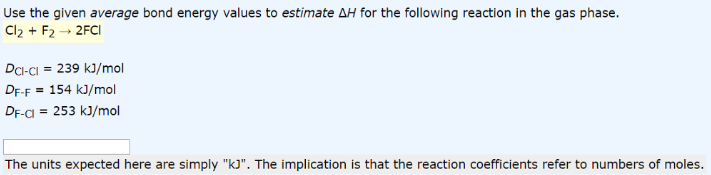# Problem: Use the given average bond energy values to estimate ΔH for the following reaction in the gas phase. Cl2 + F2 → 2FCl DCl-Cl = 239 kJ/mol DF-F = 154 kJ/mol DF-Cl = 253 kJ/mol The units expected here are simply "kJ". The implication is that the reaction coefficients refer to numbers of moles.

###### FREE Expert Solution
81% (245 ratings)###### Problem Details

Use the given average bond energy values to estimate ΔH for the following reaction in the gas phase.

Cl2 + F→ 2FCl

DCl-Cl = 239 kJ/mol

DF-F = 154 kJ/mol

DF-Cl = 253 kJ/mol

The units expected here are simply "kJ". The implication is that the reaction coefficients refer to numbers of moles.